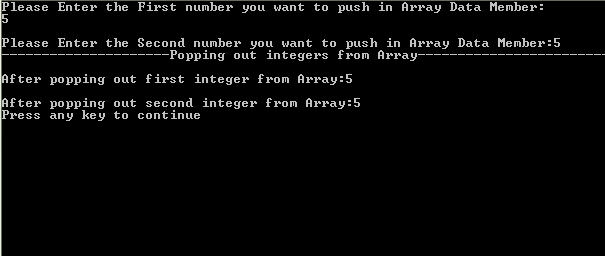# Arrays as Class Data Member

In this tutorial you will learn: 1. How to make Arrays as Class Data Member? 2. Why to use arrays as attributes of the class? 3. How to make array of objects? 4. C++ syntax Arrays can also be the data members of the class, just like integer, float and other data type integers we can also define an array as the data members. Arrays are used as data members because if the user requires that the object contain a lot of information which is to be saved in the array then he will use array as the data member of the class. It must be noted here that whenever we define array as the data member of the class we must define its size dynamically and if we don’t do so then the we have to define the array dynamically using ‘new’ keyword. Arrays as the data member of the class can be defined as follow Class myclass { private: int a; // here we write remaining part of the class }; We can also make array of objects, but this is done in main of the program. It allows us to make a lot of objects and store them in an array. By making an array of objects we can set the values of all the objects using the smaller piece of code. We define an array of objects and initialzed the value using ‘setDist’ function in the main as follow void main() { Distance dist; for (int i=0; iArray as Data Member Basic Step: Open Dev C++ then File > new > source file and start writing the code below.
`#include<iostream>#include<conio.h>using namespace std;`
These are the basic header files which are required for C++ programming. Using namespace std is used at the top of the program because if its not written then we have to write ‘std::’ with every line of the program. #include includes the C++ built in libraries and iostream is used for input and output of the program. Conio.h is used to show output on the screen, if it is not present , the screen flashes and disappears hence not showing output.
`const int MAX=10; class Stack {	int st[MAX];	int top;	public:		Stack() : top(-1) {}		void push(int var) {st[++top]=var;}		int pop() {return st[top--];}}; `
First of all we defined the size of constant integer ‘MAX’, it is the size of the Array which is the data member of the class. Then we made a class named stack. We defined two data members, one is the top and other is the array ‘st’. Next we have a constructor in which we have initialized the value of top equal to -1. Next we have a push function which takes an integer as argument and puts that argument at the top of the stack. Then we have a pop function which removes the top element of the stack and returns the value.
`void main(){	Stack s1;	int a,b;	cout<<"Please Enter the First number you want to push in Array Data Member:";	cin>>a;	s1.push(a);	cout<<"\nPlease Enter the Second number you want to push in Array Data Member:";	cin>>b;	s1.push(b);	cout<<"---------------------Popping out integers from Array------------------------\n";	cout<<"\nAfter popping out first integer from Array:"<<s1.pop()<<endl;	cout<<"\nAfter popping out second integer from Array:"<<s1.pop()<<endl;  } `
In the above code we have made an object “s1”of the class stack, then we take 2 inputs from the user and push the input value(integer) at the top of the stack one after the other. Then in the next step the elements that we pushed into the stack are popped out and displayed on the screen. strong>Constant Data Members Basic Step: Open Dev C++ then File > new > source file and start writing the code below. Execute > compile then Execute > run Output